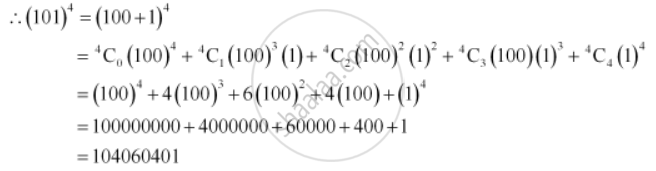CBSE (Arts) Class 11CBSE
Share
Notifications

View all notifications
Books Shortlist
Your shortlist is empty

# Using Binomial Theorem, Evaluate (101)4 - CBSE (Arts) Class 11 - Mathematics

Login
Create free account

Forgot password?
ConceptBinomial Theorem for Positive Integral Indices

#### Question

Using Binomial Theorem, evaluate (101)4

#### Solution

101 can be expressed as the sum or difference of two numbers whose powers are easier to calculate and then, Binomial Theorem can be applied.

It can be written that, 101 = 100 + 1Is there an error in this question or solution?

#### APPEARS IN

Solution Using Binomial Theorem, Evaluate (101)4 Concept: Binomial Theorem for Positive Integral Indices.
S